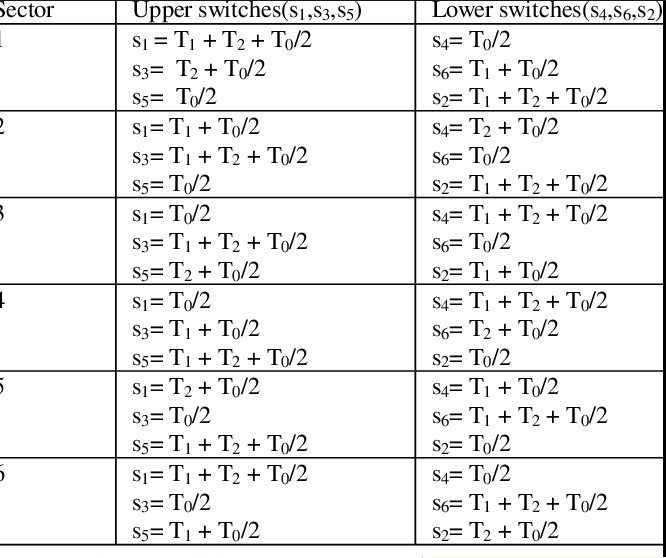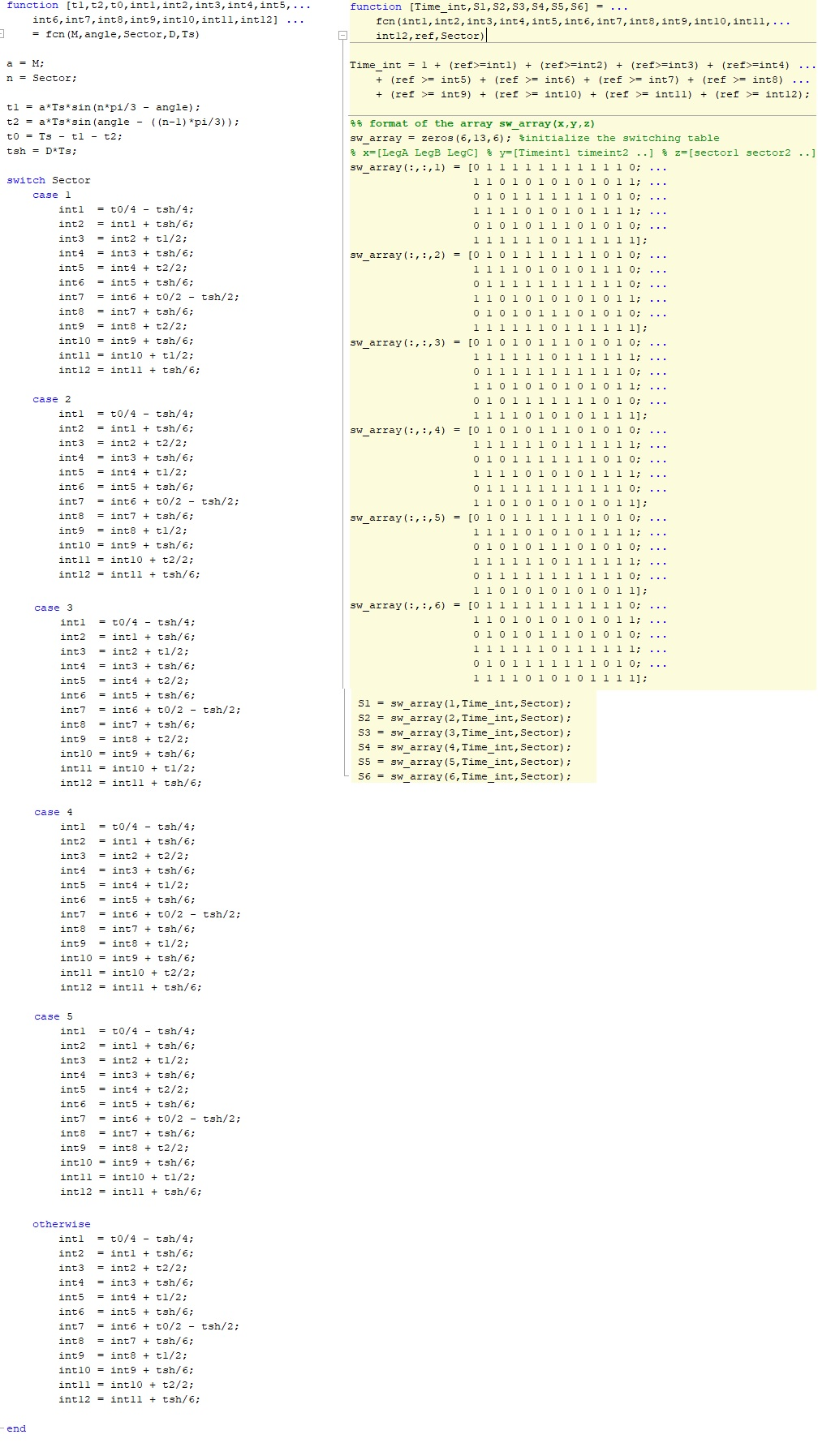# quasi z source inverter svpwm method

#### cagdas501

##### Newbie
Hello, I want to generate switching signals for a quasi z source inverter using the svpwm method. I can generate the traditional svpwm method, but I couldn't add shoot through time on it. I have researched on MathWorks and found that the code used in the image can be implemented, but I don't fully understand the code. Although I understand what it does in general, I can't adapt it to the format used in my switching time table. I would be very grateful if someone who has worked on this before or can help me on how to adapt this code to the format in my table. I have been trying to find a solution for 2 weeks.
I apologize in advance if it is forbidden to share code, but I have no other choiceHi,

With all the screenshots nobody can copy and paste text.
Makes it more hard to help than necessary (besides the increased file size).

Why not press the [ C O D E ] button and copy and paste your code/text?

Klaus

•cagdas501

Hi,

With all the screenshots nobody can copy and paste text.
Makes it more hard to help than necessary (besides the increased file size).

Why not press the [ C O D E ] button and copy and paste your code/text?

Klaus
Thanks for advice, here my codes.

Code:
function [t1,t2,t0,int1,int2,int3,int4,int5,...
int6,int7,int8,int9,int10,int11,int12] ...
= fcn(M,angle,Sector,D,Ts)

a = M;
n = Sector;

t1 = a*Ts*sin(n*pi/3 - angle);
t2 = a*Ts*sin(angle - ((n-1)*pi/3));
t0 = Ts - t1 - t2;
tsh = D*Ts;

switch Sector
case 1
int1  = t0/4 - tsh/4;
int2  = int1 + tsh/6;
int3  = int2 + t1/2;
int4  = int3 + tsh/6;
int5  = int4 + t2/2;
int6  = int5 + tsh/6;
int7  = int6 + t0/2 - tsh/2;
int8  = int7 + tsh/6;
int9  = int8 + t2/2;
int10 = int9 + tsh/6;
int11 = int10 + t1/2;
int12 = int11 + tsh/6;

case 2
int1  = t0/4 - tsh/4;
int2  = int1 + tsh/6;
int3  = int2 + t2/2;
int4  = int3 + tsh/6;
int5  = int4 + t1/2;
int6  = int5 + tsh/6;
int7  = int6 + t0/2 - tsh/2;
int8  = int7 + tsh/6;
int9  = int8 + t1/2;
int10 = int9 + tsh/6;
int11 = int10 + t2/2;
int12 = int11 + tsh/6;

case 3
int1  = t0/4 - tsh/4;
int2  = int1 + tsh/6;
int3  = int2 + t1/2;
int4  = int3 + tsh/6;
int5  = int4 + t2/2;
int6  = int5 + tsh/6;
int7  = int6 + t0/2 - tsh/2;
int8  = int7 + tsh/6;
int9  = int8 + t2/2;
int10 = int9 + tsh/6;
int11 = int10 + t1/2;
int12 = int11 + tsh/6;

case 4
int1  = t0/4 - tsh/4;
int2  = int1 + tsh/6;
int3  = int2 + t2/2;
int4  = int3 + tsh/6;
int5  = int4 + t1/2;
int6  = int5 + tsh/6;
int7  = int6 + t0/2 - tsh/2;
int8  = int7 + tsh/6;
int9  = int8 + t1/2;
int10 = int9 + tsh/6;
int11 = int10 + t2/2;
int12 = int11 + tsh/6;

case 5
int1  = t0/4 - tsh/4;
int2  = int1 + tsh/6;
int3  = int2 + t1/2;
int4  = int3 + tsh/6;
int5  = int4 + t2/2;
int6  = int5 + tsh/6;
int7  = int6 + t0/2 - tsh/2;
int8  = int7 + tsh/6;
int9  = int8 + t2/2;
int10 = int9 + tsh/6;
int11 = int10 + t1/2;
int12 = int11 + tsh/6;

otherwise
int1  = t0/4 - tsh/4;
int2  = int1 + tsh/6;
int3  = int2 + t2/2;
int4  = int3 + tsh/6;
int5  = int4 + t1/2;
int6  = int5 + tsh/6;
int7  = int6 + t0/2 - tsh/2;
int8  = int7 + tsh/6;
int9  = int8 + t1/2;
int10 = int9 + tsh/6;
int11 = int10 + t2/2;
int12 = int11 + tsh/6;

end

function [Time_int,S1,S2,S3,S4,S5,S6] = ...
fcn(int1,int2,int3,int4,int5,int6,int7,int8,int9,int10,int11,...
int12,ref,Sector)

Time_int = 1 + (ref>=int1) + (ref>=int2) + (ref>=int3) + (ref>=int4) ...
+ (ref >= int5) + (ref >= int6) + (ref >= int7) + (ref >= int8) ...
+ (ref >= int9) + (ref >= int10) + (ref >= int11) + (ref >= int12);

%% format of the array sw_array(x,y,z)
sw_array = zeros(6,13,6); %initialize the switching table
% x=[LegA LegB LegC] % y=[Timeint1 timeint2 ..] % z=[sector1 sector2 ..]
sw_array( :,:,1) = [0 1 1 1 1 1 1 1 1 1 1 1 0; ...
1 1 0 1 0 1 0 1 0 1 0 1 1; ...
0 1 0 1 1 1 1 1 1 1 0 1 0; ...
1 1 1 1 0 1 0 1 0 1 1 1 1; ...
0 1 0 1 0 1 1 1 0 1 0 1 0; ...
1 1 1 1 1 1 0 1 1 1 1 1 1];
sw_array( :,:,2) = [0 1 0 1 1 1 1 1 1 1 0 1 0; ...
1 1 1 1 0 1 0 1 0 1 1 1 0; ...
0 1 1 1 1 1 1 1 1 1 1 1 0; ...
1 1 0 1 0 1 0 1 0 1 0 1 1; ...
0 1 0 1 0 1 1 1 0 1 0 1 0; ...
1 1 1 1 1 1 0 1 1 1 1 1 1];
sw_array( :,:,3) = [0 1 0 1 0 1 1 1 0 1 0 1 0; ...
1 1 1 1 1 1 0 1 1 1 1 1 1; ...
0 1 1 1 1 1 1 1 1 1 1 1 0; ...
1 1 0 1 0 1 0 1 0 1 0 1 1; ...
0 1 0 1 1 1 1 1 1 1 0 1 0; ...
1 1 1 1 0 1 0 1 0 1 1 1 1];
sw_array( :,:,4) = [0 1 0 1 0 1 1 1 0 1 0 1 0; ...
1 1 1 1 1 1 0 1 1 1 1 1 1; ...
0 1 0 1 1 1 1 1 1 1 0 1 0; ...
1 1 1 1 0 1 0 1 0 1 1 1 1; ...
0 1 1 1 1 1 1 1 1 1 1 1 0; ...
1 1 0 1 0 1 0 1 0 1 0 1 1];
sw_array( :,:,5) = [0 1 0 1 1 1 1 1 1 1 0 1 0; ...
1 1 1 1 0 1 0 1 0 1 1 1 1; ...
0 1 0 1 0 1 1 1 0 1 0 1 0; ...
1 1 1 1 1 1 0 1 1 1 1 1 1; ...
0 1 1 1 1 1 1 1 1 1 1 1 0; ...
1 1 0 1 0 1 0 1 0 1 0 1 1];
sw_array( :,:,6) = [0 1 1 1 1 1 1 1 1 1 1 1 0; ...
1 1 0 1 0 1 0 1 0 1 0 1 1; ...
0 1 0 1 0 1 1 1 0 1 0 1 0; ...
1 1 1 1 1 1 0 1 1 1 1 1 1; ...
0 1 0 1 1 1 1 1 1 1 0 1 0; ...
1 1 1 1 0 1 0 1 0 1 1 1 1];

S1 = sw_array(1,Time_int,Sector);
S2 = sw_array(2,Time_int,Sector);
S3 = sw_array(3,Time_int,Sector);
S4 = sw_array(4,Time_int,Sector);
S5 = sw_array(5,Time_int,Sector);
S6 = sw_array(6,Time_int,Sector);
if it its needed;
function [phaseA,phaseB, phaseC, alpha, beta, z, Mag, ang, Sec] = fcn (Amp,Freq)
%function [phaseA,phaseB, phaseC, alpha, beta, Mag, ang, Sec, z] = fcn (Amp,Freq)

%% generate 3 phase sine waveform
% multiply amplitude with gain

Amp1 = Amp*0.1;
phaseA = Amp1*sin(Freq);
phaseB = Amp1*sin(Freq + 2*pi/3);
phaseC = Amp1*sin(Freq + 4*pi/3);

%% Convert 3 phase to ab
alphaT = [1      -1/2          -1/2];
betaT  = [0    sqrt(3)/2    -sqrt(3)/2];

U = [phaseA; phaseB; phaseC];

alpha = 2/3*alphaT*U;
beta  = 2/3*betaT*U;

% Sector Selection
% function [ Mag, ang, Sec, z] = fcn(alpha,beta)
%convert to polar format
%z=u(1) + 1i*u(2);

z = alpha + 1i*beta;
Mag = abs(z);
ang = atan2(imag(z),real(z));
alpha1=ang*180/pi;

% Sec = (alpha1 >= 45 & alpha1 < 135)*2 + (alpha1 >=135 & alpha1 < 180)*3 + (alpha1 >=-180 & alpha1 < -135)*3 +(alpha1 >=-135 & alpha1 < -45)*4 ...
%     +(alpha1 >= -45 & alpha1 < 45)*1;
Sec = (alpha1 >= 0 & alpha1 < 60)*1 + (alpha1 >= 60 & alpha1 < 120)*2 + (alpha1 >= 120 & alpha1 < 180)*3 ...
+ (alpha1 >= -180 & alpha1 < -120)*4 + (alpha1 >= -120 & alpha1 < -60)*5 + (alpha1 >= -60 & alpha1 < 0)*6;
%(amplitude 1, frequency 50)

Last edited by a moderator:

##Commands Quick-Menu: